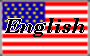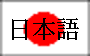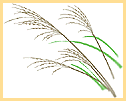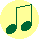#\$B7n8+(B(\$B\$D\$-\$_(B) - 9\$B7n\$NK~7n(BAn easier version in Japanese is here.

\$B%"%8%"<~JU\$K\$O!"7n\$r\$a\$G\$k=,47\$,\$"\$j\$^\$9!#F|K\\$G\$b!"B@1"Nq\$N(B8\$B7n(B15\$BF|(B(\$BB@(B \$BM[Nq\$G\$O(B9\$B7n\$NK~7n\$NF|(B)\$B!"K~7n\$r\$J\$,\$a\$^\$9!#\$3\$N=,47\$O\$*7n8+\$H8F\$P\$l\$F\$*(B \$B\$j!"\$=\$b\$=\$b!"G@IW\$?\$A\$,K-:n\$r5'\$C\$?Iw=,\$+\$i;O\$^\$j\$^\$9!#(B\$B\$9\$9\$-(B
\$B!!(B
\$B7n8+CD;R\$d!"0rN`!"5(@a\$N2LJ*!"\$9\$9\$-!"Gk\$J\$I\$rAk:]\$KCV\$-!"K~7n\$K6!\$(\$^(B \$B\$9!#(B\$B\$3\$N%\$%Y%s%H\$N\$3\$H\$r2N\$C\$?\$H\$F\$bM-L>\$G4JC1\$J(B "\$B\$D\$-(B " \$B\$H!"(B " \$B\$&\$5\$.(B"\$B\$H\$\$\$&2N\$,\$"\$j\$^\$9!#\$<\$RJ9\$\$(B \$B\$F\$_\$F\$/\$@\$5\$\$!#(B